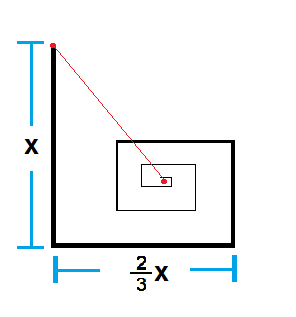# The odd theoretical ant

Algebra Level 2An ant travels along a straight path with a distance of $x$ meters. From then on, it turns left and covers $\frac{2}{3}$ of the straight distance it traveled before turning, and continues doing this until it eventually reaches an unknown point $P$ (referred to as the red point in the middle of the picture). Refer to the figure above.

The distance of $P$ from the ant's starting point is $kx$ meters, where $k$ is a positive constant which can be expressed in the form $\frac{a \sqrt {b}}{c}$, where $\gcd(a,c) = 1$ and $b$ is square free. Determine the value of $a + b + c$.

Challenge: Determine an explicit formula for $k$.

×